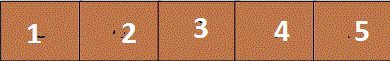## Jun 17, 2013# Find minimum element in rotated and sorted array.

Problem : We have to find an element which is having lowest value in the given array which is sorted and rotated. FYI: This question was asked me in oracle interview.(All elements of the array are unique)
what is sorted and rotated array ?
Sorted array (ONLY) :

Sorted and rotated array (BOTH):

By brute force approach we can find that element in O(n) , off course this is not expected answer
any interviewer would like to hear at least not in F2F interview  :P
So what next ?  Since we have a sorted array  and we have to find a solution better than O(n) so Binary search may be handy to solve this problem. If we see diagram closely we can find that  main rationale behind this problem is :
`````` If (array is rotated )
lowest element is that element whose previous element is always higher
else
first element is lowest element.  ``````
Algorithm :
``````  Do simple binary search
find middleIndex from startIndex and endIndex of array
if (element@middleIndex > element@(middleIndex+1))
lowest element is element@(middle+1)
return "we are done!!"
else if (element@middleIndex < element@(middleIndex-1))
lowest element is element@(middle)
return "we are done!!"
else
if(element@middleIndex < element@endIndex)
Do not move in right direction , since elements are in increasing order.
go to left direction and  repeat binary search methd
else
Do not move in left direction , since elements are in increasing order.
go to right direction and  repeat binary search methd
``````
Now we will write the sample java code for this .
`````` public class FindMinimumInRotatedArray {
public FindMinimumInRotatedArray() {
super();
}
private int findMinInSortedAndRotated(int arr[], int low, int high)
{
// Added to handle the case when array is not
// rotated at all
if (high < low) return arr;
// If there is only one element left
if (high == low) return arr[low];
// Find mid
int mid = low + (high - low)/2; /*To avoid overflow do not use (low + high)/2;*/
if (mid < high && arr[mid+1] < arr[mid])
return arr[mid+1];
// Check if mid itself is minimum element
if (mid > low && arr[mid] < arr[mid - 1])
return arr[mid];
// Decide whether we need to go to left half or right half
if (arr[high] > arr[mid])
return findMin(arr, low, mid-1);
return findMin(arr, mid+1, high);
}
public static void main(String[] args) {
FindMinimumInRotatedArray findMinimumInRotatedArray = new FindMinimumInRotatedArray();
int[] arr = { 6, 7, 8, 9, 1, 2, 3, 4, 5 };
System.out.println(findMinimumInRotatedArray.findMinInSortedAndRotated(arr, 0, 9));
}
}  ``````
Sample output : 1

when we execute program we will get 1 output , since it is minimum element.

Now if we twist the question little bit what will happen if all elements of the array are not unique.
-------------------------------------------------------------------------------------------------------
Happy learning!!
Nikhil

#### 1 comment:

1.